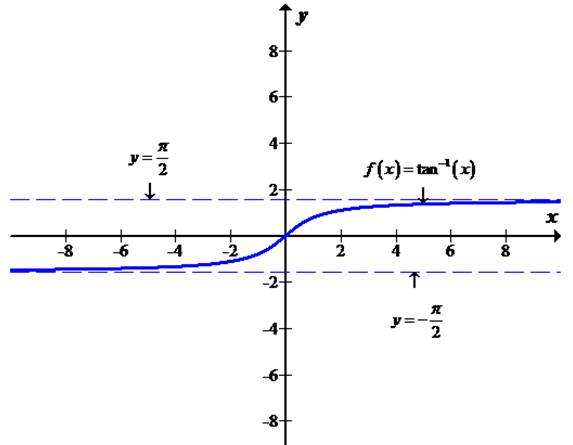# Whether the statement “A function can have two different horizontal asymptotes” is true or false.### Single Variable Calculus: Concepts...

4th Edition
James Stewart
Publisher: Cengage Learning
ISBN: 9781337687805### Single Variable Calculus: Concepts...

4th Edition
James Stewart
Publisher: Cengage Learning
ISBN: 9781337687805

#### Solutions

Chapter 2, Problem 9RQ
To determine

## To find: Whether the statement “A function can have two different horizontal asymptotes” is true or false.

Expert Solution

The statement “A function can have two different horizontal asymptotes” is true.

### Explanation of Solution

Given information:

The given statement is “A function can have two different horizontal asymptotes”.

Calculation:

The horizontal asymptote is a horizontal tangent line to the curve at infinity. The line y=L is called a horizontal asymptote of the curve y=f(x) if either limxf(x)=L or limxf(x)=L .

Consider the function

f(x)=tan1(x)

Calculate the value of limxf(x)

limxf(x)=limxtan1(x)=π2

Hence, y=π2 is a horizontal asymptote to the curve.

Calculate the another value of limxf(x)

limxf(x)=limxtan1(x)=π2

Hence, y=π2 is another horizontal asymptote to the curve.

These results are observed from the below graph.Therefore, the statement “A function can have two different horizontal asymptotes” is true.

### Have a homework question?

Subscribe to bartleby learn! Ask subject matter experts 30 homework questions each month. Plus, you’ll have access to millions of step-by-step textbook answers!